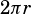How does the arc length equal the radian measure of angle AOB ? (question 2 on page 271)

When a circle’s radius is 1, its arc lengths are equal to their radian measures.

The full circumference of a circle is. When, the circumference is—the same as the radian measure of a full circle.

Remember that arc length and central angle are proportional:Whenand the circumference is, the denominators in that equation are equal, so the arc length will always equal the measure of the central angle in radians.